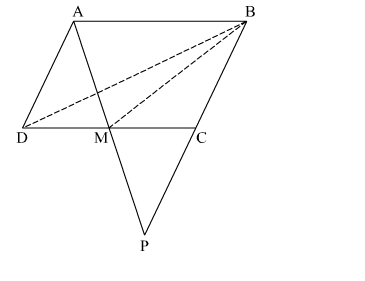# ABCD is a parallelogram in which BC is produced to P such that CP = BC, as shown in the adjoining figure.

Question:

ABCD is a parallelogram in which BC is produced to P such that CP BC, as shown in the adjoining figure. AP intersects CD at M. If ar(DMB) = 7 cm2, find the area of parallelogram ABCD.Solution:

In $\triangle \mathrm{MDA}$ and $\triangle \mathrm{MCP}$,

$\angle \mathrm{DMA}=\angle \mathrm{CMP}$         (Vertically opposite angles)

$\angle \mathrm{MDA}=\angle \mathrm{MCP} \quad$ (Alternate interior angles)

So, $\triangle \mathrm{MDA} \cong \triangle \mathrm{MCP} \quad$ (ASA congruency)

$\Rightarrow \mathrm{DM}=\mathrm{MC} \quad(\mathrm{CPCT})$

$\Rightarrow \mathrm{M}$ is the midpoint of $\mathrm{DC}$

$\Rightarrow B M$ is the median of $\triangle B D C$

$\Rightarrow \operatorname{ar}(\triangle B M C)=\operatorname{ar}(\triangle D M B)=7 \mathrm{~cm}^{2}$

$\Rightarrow \operatorname{ar}(\triangle B M C)=\operatorname{ar}(\triangle D M B)=7 \mathrm{~cm}^{2}$

$\operatorname{ar}(\triangle B M C)+\operatorname{ar}(\triangle D M B)=\operatorname{ar}(\triangle D B C)=7+7=14 \mathrm{~cm}^{2}$

Area of parallelogram $\mathrm{ABCD}=2 \times \operatorname{ar}(\triangle \mathrm{DBC})=2 \times 14=28 \mathrm{~cm}^{2}$